Learning Library
Who we are
Guidance

# 5.NF.B.5 Worksheets, Workbooks, Lesson Plans, and Games

#### CCSS.MATH.CONTENT.5.NF.B.5

:
"Interpret multiplication as scaling (resizing), by:"

These worksheets, lesson plans, and exercises can help students practice this Common Core State Standards skill.

## Worksheets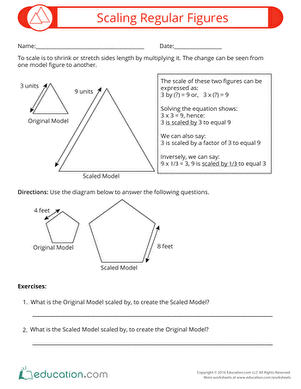Scaling Regular Figures
Worksheet
Scaling Regular Figures
How much bigger is this shape? Or how much smaller? Get your students thinking and scaling regular two-dimensional figures using this printable worksheet.
Math
Worksheet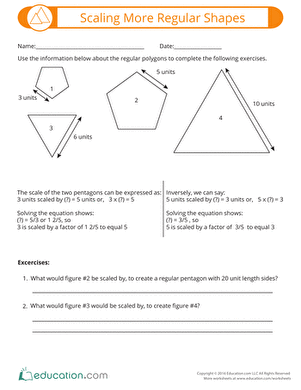Scaling More Regular Shapes
Worksheet
Scaling More Regular Shapes
Scaling shapes is a gret way to apply multiplication concepts to geometry. Use this resource to show your students how to draft regular two-dimensional figures to scale.
Math
Worksheet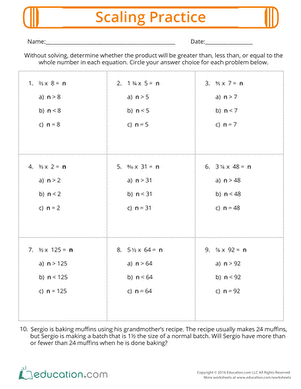Scaling Practice
Worksheet
Scaling Practice
Give your students some practice scaling numbers when multiplying a whole number and a fraction.
Math
Worksheet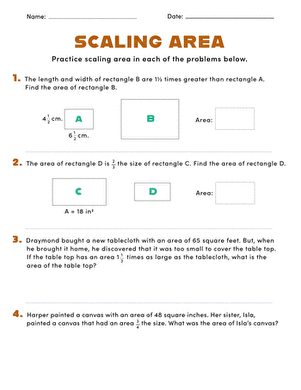Scaling Area
Worksheet
Scaling Area
Challenge your students to think about multiplication as scaling in these exercises that involve area.
Math
Worksheet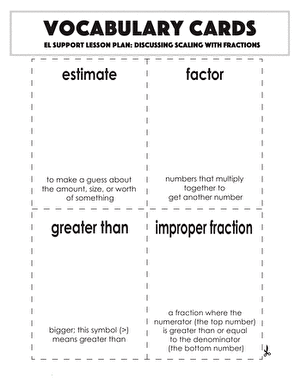Vocabulary Cards: Discussing Scaling with Fractions
Worksheet
Vocabulary Cards: Discussing Scaling with Fractions
Use these vocabulary cards with the EL Support Lesson: Discussing Scaling with Fractions.
Math
Worksheet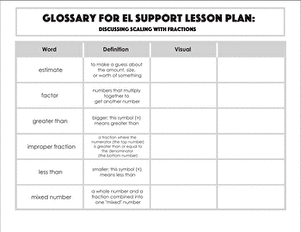Glossary: Discussing Scaling with Fractions
Worksheet
Glossary: Discussing Scaling with Fractions
Use this glossary with the EL Support Lesson: Discussing Scaling with Fractions.
Math
Worksheet

## Lesson Plans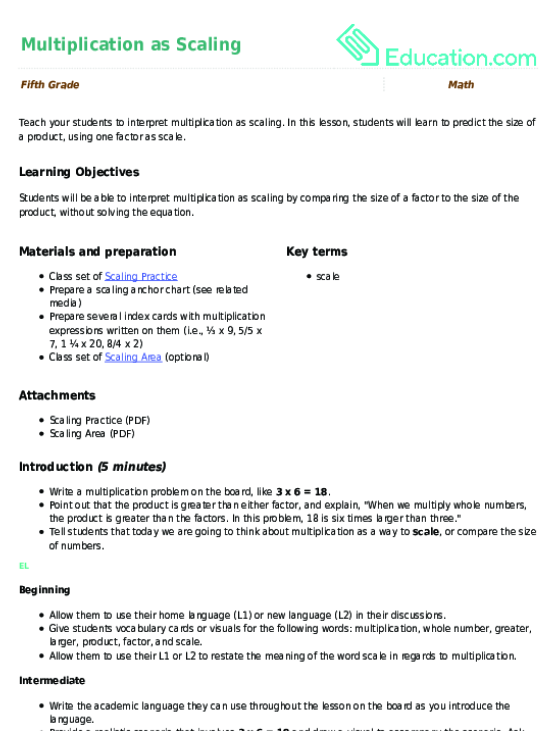Multiplication as Scaling
Lesson plan
Multiplication as Scaling
Teach your students to interpret multiplication as scaling. In this lesson, students will learn to predict the size of a product, using one factor as scale.
Math
Lesson plan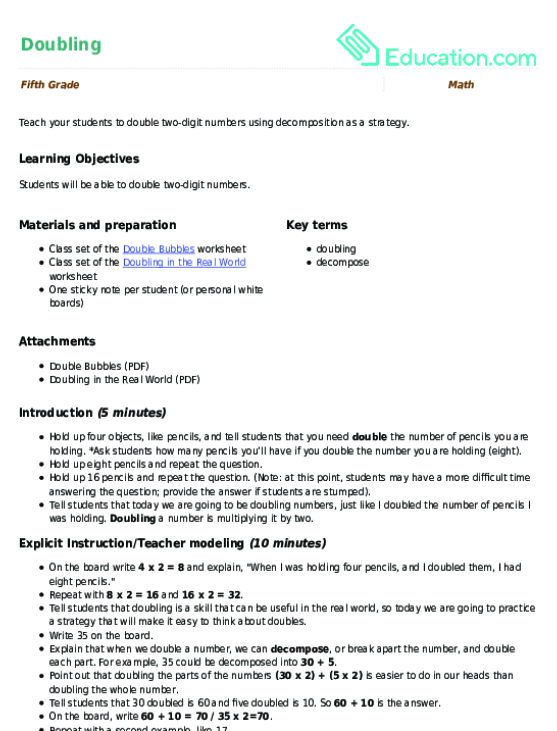Doubling
Lesson plan
Doubling
Teach your students to double two-digit numbers using decomposition as a strategy.
Math
Lesson plan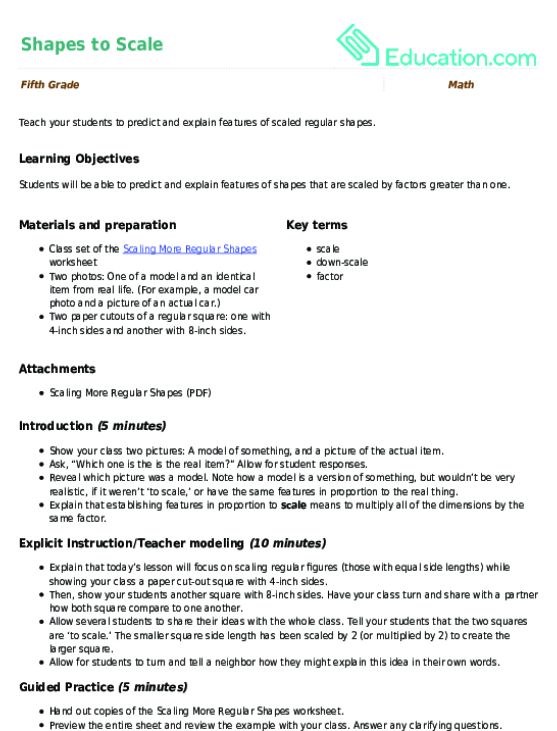Shapes to Scale
Lesson plan
Shapes to Scale
Teach your students to predict and explain features of scaled regular shapes.
Math
Lesson plan

## Workbooks

No workbooks found for this common core node.

## Games

No games found for this common core node.

## Exercises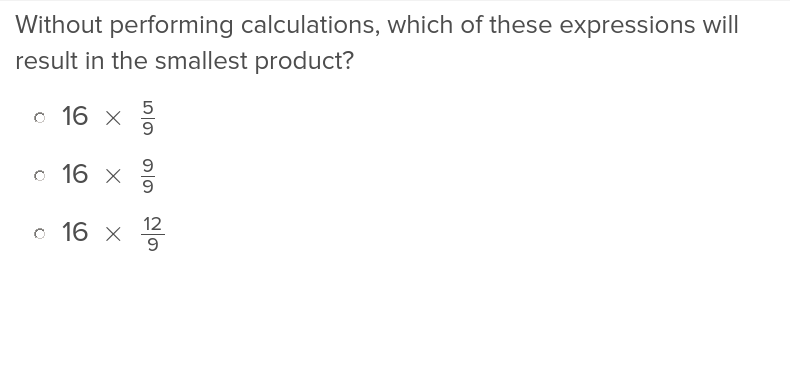Interpret Multiplication of Fractions as Scaling
Exercise
Interpret Multiplication of Fractions as Scaling
Introduce your fifth graders to multiplying by fractions with these exercises that help them understand the effect of numerators and denominators of equations.